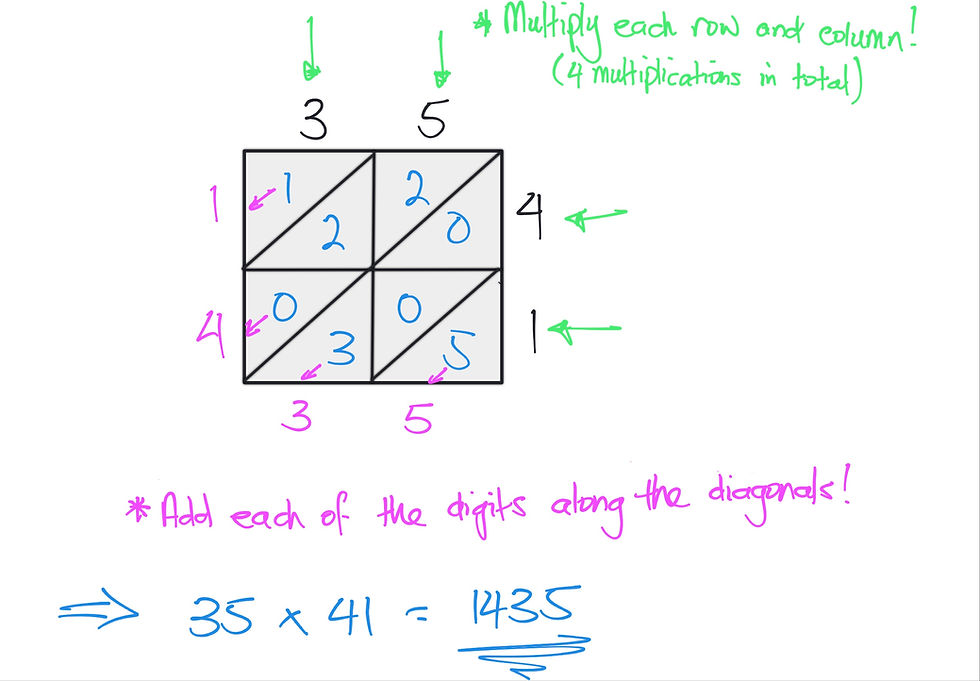top of page
Search

# A Brief History of Multiplication Algorithms

The history of multiplication algorithms dates back to ancient times when people used various methods to multiply numbers. One of the earliest multiplication algorithms was the Egyptian multiplication method, which involved repeated doubling and halving of numbers. This method was used by ancient Egyptians around 3000 BCE. Here is an example of how this method works:Another early multiplication algorithm was the lattice method, which was used in India around the 12th century. This method involves drawing a lattice or grid and placing the digits of the two numbers being multiplied in the rows and columns. Then, the products of each digit are placed in the corresponding box, and the final product is obtained by summing the numbers along the diagonals.In the 16th century, the Italian mathematician Fibonacci introduced the Hindu-Arabic numeral system to Europe, which allowed for more efficient multiplication algorithms. The lattice method was modified and simplified in Europe and became known as the "Italian method."

In the 17th century, the German mathematician Gottfried Wilhelm Leibniz developed a more efficient algorithm for multiplication, which is now known as the "grid method" or "Leibniz method." This method involves breaking down each number into its place values and then multiplying each digit by every digit in the other number, adding up the results along the way.The traditional long multiplication algorithm that is taught in schools today was developed in the 19th century and is based on the grid method. It involves breaking down the numbers into their place values, multiplying each digit in one number by each digit in the other number, and then adding up the results to obtain the final product.

The implementation of multiplication algorithms into mainstream education began in the 19th century when formal schooling became more widespread. At first, multiplication was taught through rote memorisation of multiplication tables. However, as the need for more efficient methods of multiplication grew, the traditional long multiplication algorithm was introduced into the curriculum.

Today, we recognise the need for a more conceptual approach to teaching multiplication, and many of the early multiplication skills are taught using arrays. Multiplication arrays elaborate on the grid method and provide a visual scaffold for all of the aforementioned methods. It is important to note that although each of these algorithms can be appropriately used for finding a product of two factors, they are all still a process and as such require rote memorisation of the steps to complete.

So, my advice when incorporating any of these methods is to tread cautiously and do so only after the conceptual understanding has been developed. They are fun to work with and can make great lessons, but if students don't know why they work, then they will only add to the cognitive load of students and result in poor application and performance once the problems being solved become more complex.

If you loved this post and would like to know more, why not reach out and book a chat here or just email me at nadia@emmaths.com.au . I'd love to hear from you!

References

• Boyer, C. B., & Merzbach, U. C. (2011). A History of Mathematics (3rd ed.). Wiley

• Wrigley, Andrew, 2016, Mathemagical multiplication, Vinculum, vol:53, issue 2, pp 14